# Difference between revisions of "Cauchy-Kovalevskaya theorem"

A theorem stating that the Cauchy problem has a (unique) analytic solution locally if the functions occurring in the differential equation (or system of differential equations) and all the initial data, together with the non-characteristic initial surface, are analytic.

For a system ofpartial differential equations inunknown functions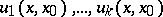, of the form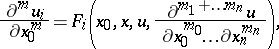(1)

where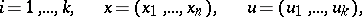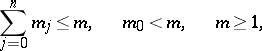the Cauchy–Kovalevskaya theorem states the following: The Cauchy problem posed by the initial data(2)

where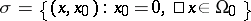is the initial surface of the data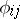, has a unique analytic solution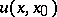in some domainin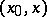-space containing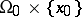, if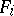andare analytic functions of all their arguments.

Consider a linear system of differential equations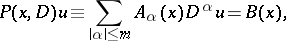(3)

whereis a vector with non-negative integer coordinates;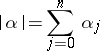is the order of the differential operator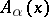,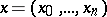, is a given square matrix of order;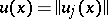,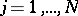, is the column-vector of unknown functions; and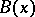is a given-vector.

Generally speaking, the Cauchy–Kovalevskaya theorem does not exclude the possibility of the Cauchy problem having non-analytic solutions in addition to the analytic solution. However, the Cauchy problem for a linear system (3) with analytic coefficientsand Cauchy conditions on an analytic non-characteristic surfacehas at most one solution in a certain neighbourhood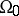of. This assertion is valid without any analyticity assumptions concerning the initial data and the solution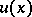.

A solution of the Cauchy problem (1), (2), the existence of which is guaranteed by the Cauchy–Kovalevskaya theorem, may turn out to be unstable (since a small variation of the initial data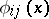may induce a large variation of the solution). For example, this is the case when the system (1) is of elliptic type. If the initial data are non-analytic, the Cauchy problem (1), (2) may become meaningless unless attention is confined to hyperbolic systems (1).

For a broad class of equations, the Cauchy–Kovalevskaya theorem may be generalized to the case in which the initial manifold is characteristic at every point (see , ). In that case the initial functions cannot be prescribed at will; they must satisfy certain conditions, dictated by the differential equation.

A characteristic Cauchy problem (cf. Cauchy characteristic problem) may have more than one solution. In particular, one has the following proposition. Let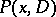be a differential equation of orderwith principal part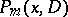and with real-analytic coefficients, defined in a neighbourhoodof a point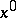of the Euclidean space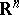, and letbe a real-analytic function insuch that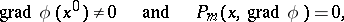but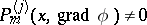at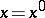for some. Then there exists a neighbourhoodofand a functionof class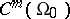, analytic whenever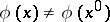, such that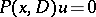and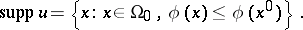If the initial manifold is characteristic along certain curves then, generally speaking, the solution of the characteristic Cauchy problem is non-unique in some neighbourhood of the initial surface and the degree of bifurcation is defined by the geometrical nature of the corresponding characteristic surfaces. The theorem was first proved by S.V. Kovalevskaya (1875).

How to Cite This Entry:
Cauchy-Kovalevskaya theorem. Encyclopedia of Mathematics. URL: http://encyclopediaofmath.org/index.php?title=Cauchy-Kovalevskaya_theorem&oldid=11505
This article was adapted from an original article by A.M. Nakhushev (originator), which appeared in Encyclopedia of Mathematics - ISBN 1402006098. See original article